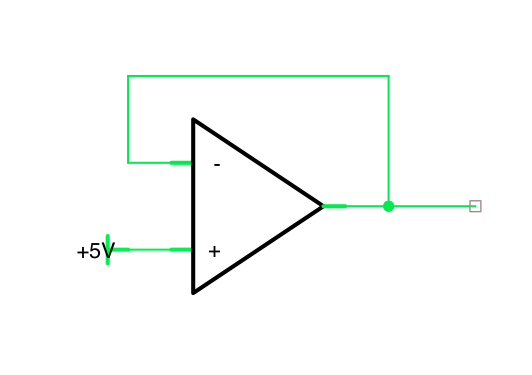# What is an Operational Amplifier?

An operational amplifier, often known as an opamp (operational amplifier) ​​is a DC-coupled high gain electronic amplifier device that has two inputs and one output. In this configuration, the output of the device is generally hundreds of thousands of times greater than the potential difference between its inputs.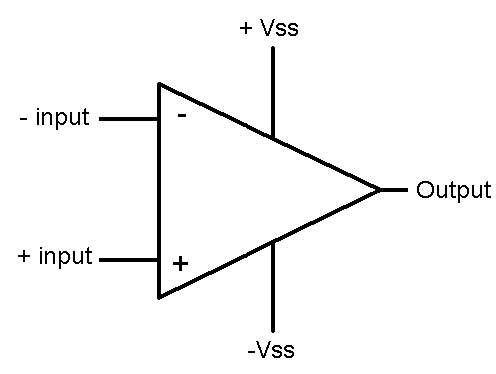## An operational amplifier only serves to amplify?

Operational amplifiers have many other uses apart from amplifying such as:

### Signal conditioning

Increase its power in addition to its intensity so that it does not suffer distortion or attenuation by the measurement process, especially if the input impedance of the sensor circuit is not high enough, this is achieved by ensuring that the signal to be measured has a minimum level of power delivered; conditioning also includes:

• Step to a logarithmic scale.
• Change offset.
• Polarity.
• Modulation.
• Greater immunity to noise.
• Stability.

### Impedance matching

Although it can be considered part of the conditioning, it is more general to the isolation of circuits.

### Active filters

Go high. They only let pass frequencies that are below a certain frequency.

It passes casualties. They only let pass those frequencies that are greater than a certain frequency.

Passband. They let pass a certain group of frequencies that are within the range of the filter.

Reject band. Does not allow frequencies between the upper and lower cutoff frequencies to pass

### Oscillator circuits

Pulse and waveform generators.

### Analog signal processing.

• Comparators.
• Integrators.
• Derivatives.
• Delay elements.
• Phase changes.
• Rectifiers.

## Logical signal processing.

In certain applications, the same effect is obtained from digital logic gates, providing either a zero (false) or saturation (true or “logical one”) voltage value.

## How is an op-amp?

The opamp 741 has 5 connection pins; inverter, non-inverter, a positive voltage, a negative voltage, output.## Basic Operational Amplifier Settings.

Operational amplifiers can be connected according to two basic amplifier circuits: (1) inverter and (2) non-inverter configurations. Almost all other op-amp circuits are based, in some way, on these two basic configurations. In addition, there are closely related variations of these two circuits, plus another basic circuit that is a combination of the first two: the differential amplifier.

### Inverting Amplifier

The basic configuration of the AO. The inverting amplifier. In this circuit, the input (+) is grounded, and the signal is applied to the input (-) through R1, with feedback from the output through R2.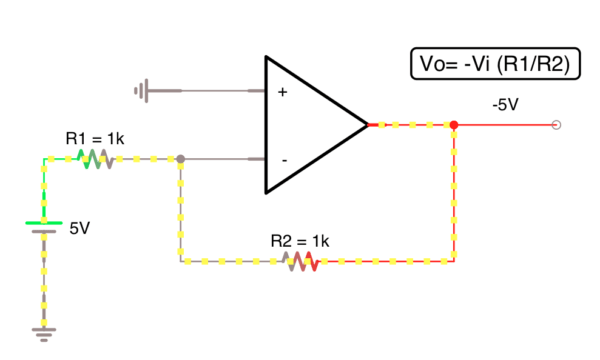### Non-inverting amplifier

In this circuit, the voltage Vi is applied to the input (+), and a fraction of the output signal, Vo, is applied to the input (-) through the voltage divider R1 – R2. Since no input, current flows at an input terminal, and since Vd = 0, the voltage at R1 will be equal to Vi.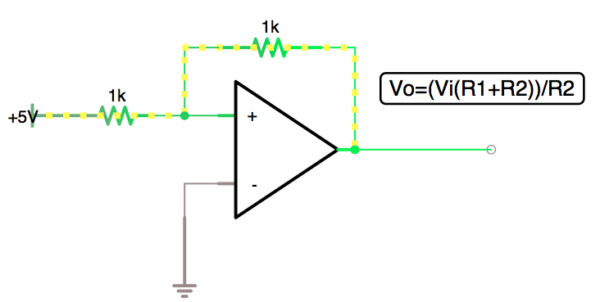Configurations based on the inverter and non-inverter circuits

### Differential amplifier.

A third configuration of the AO known as the differential amplifier is a combination of the two previous configurations. Although based on the other two circuits, the differential amplifier has unique characteristics. This circuit has signals applied to both input terminals and uses the natural differential amplification of the op-amp.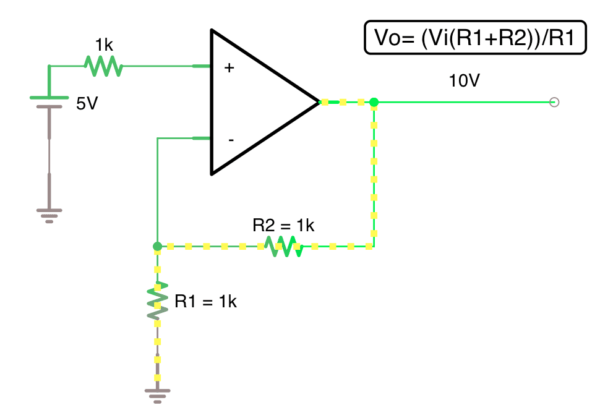Using the virtual earth characteristic in the sum (-) node of the inverting amplifier, a useful modification is obtained, the inverting adder
In this circuit, as in the inverting amplifier, the voltage V (+) is connected to ground, so the voltage V (-) will be at a virtual mass, and since the input impedance is infinite, all current I1 will circulate at via RF and we’ll call it I2. What happens, in this case, is that the current I1 is the algebraic sum of the currents provided by V1, V2 and V3.### The integrator

Both basic AO configurations have been shown to act to constantly maintain the feedback current, IF equal to IIN.
A modification of the inverting amplifier, the integrator, takes advantage of this feature. An input voltage VIN is applied to RG, which gives rise to an IIN current.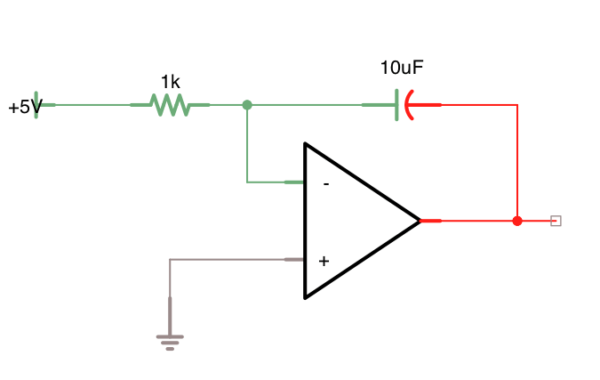### The differentiator

A second modification to the inverting amplifier, which also harnesses the current in a capacitor is the differentiator.
In this circuit, the position of R and C are the opposite of that of the integrator, the capacitive element being in the input network. Then the input current obtained is proportional to the rate of variation of the input voltage:### The voltage follower

A special modification of the non-inverting amplifier is the gain stage.
In this circuit, the input resistance has been increased to infinity, and RF is zero, and the feedback is 100%. V0 is then exactly equal to Vi, since Es = 0. The circuit is known as an “emitter follower” since the output is a phase replica with unity gain of the input voltage. The input impedance of this stage is also infinite.